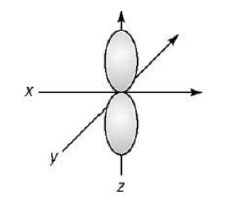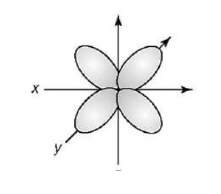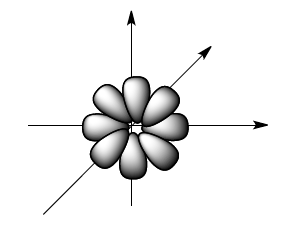Ch.7 - Quantum MechanicsWorksheetSee all chapters
 Ch.1 - Intro to General Chemistry 2hrs & 53mins 0% complete Worksheet Ch.2 - Atoms & Elements 2hrs & 49mins 0% complete Worksheet Ch.3 - Chemical Reactions 3hrs & 25mins 0% complete Worksheet BONUS: Lab Techniques and Procedures 1hr & 38mins 0% complete Worksheet BONUS: Mathematical Operations and Functions 47mins 0% complete Worksheet Ch.4 - Chemical Quantities & Aqueous Reactions 3hrs & 30mins 0% complete Worksheet Ch.5 - Gases 3hrs & 47mins 0% complete Worksheet Ch.6 - Thermochemistry 2hrs & 28mins 0% complete Worksheet Ch.7 - Quantum Mechanics 2hrs & 35mins 0% complete Worksheet Ch.8 - Periodic Properties of the Elements 1hr & 57mins 0% complete Worksheet Ch.9 - Bonding & Molecular Structure 2hrs & 5mins 0% complete Worksheet Ch.10 - Molecular Shapes & Valence Bond Theory 1hr & 31mins 0% complete Worksheet Ch.11 - Liquids, Solids & Intermolecular Forces 3hrs & 40mins 0% complete Worksheet Ch.12 - Solutions 2hrs & 17mins 0% complete Worksheet Ch.13 - Chemical Kinetics 2hrs & 22mins 0% complete Worksheet Ch.14 - Chemical Equilibrium 2hrs & 26mins 0% complete Worksheet Ch.15 - Acid and Base Equilibrium 4hrs & 42mins 0% complete Worksheet Ch.16 - Aqueous Equilibrium 3hrs & 48mins 0% complete Worksheet Ch. 17 - Chemical Thermodynamics 1hr & 44mins 0% complete Worksheet Ch.18 - Electrochemistry 2hrs & 58mins 0% complete Worksheet Ch.19 - Nuclear Chemistry 1hr & 33mins 0% complete Worksheet Ch.20 - Organic Chemistry 3hrs 0% complete Worksheet Ch.22 - Chemistry of the Nonmetals 2hrs & 1min 0% complete Worksheet Ch.23 - Transition Metals and Coordination Compounds 1hr & 54mins 0% complete Worksheet

# End of Chapter 7 Problems

See all sections

Test your skills on the following problems.

###### Emission vs. Absorption

Example #1: Which of the following transitions (in a hydrogen atom) represent emission of the smallest or shortest wavelength?

a)  n = 4 to n = 2

b)  n = 3 to n= 4

c)  n = 1 to n = 2

d)  n = 7 to n = 5

e)  n = 2 to n = 5

Example #2: Which of the following transitions represent absorption of a photon with the highest frequency?

a)  n = 3 to n = 1

b)  n = 2 to n = 4

c)  n = 1 to n =2

d)  n = 6 to n = 3

e)  n = 1 to n = 3

###### Quantum Numbers

Example #3: Provide the n, l and ml value for each of the given orbitals.

a) 7s      n =  ?                             b) 5d     n =  ?

l  =   ?                                         l =  ?

ml =   ?                                        ml =  ?

c) 2p     n =    ?                            d) 4f      n =  ?

l  =   ?                                          l  =   ?

ml =     ?                                      ml =  ?

Example #4: Which statement about the four quantum numbers is false?

a)  n = principal quantum number,            n = 1 to infinity

b)  l = azimuthal quantum number,           l = 0,1,2, . . ., (n+1)

c)  mL = magnetic quantum number, mL = (-l), . . .,0,. . ., (+l)

d)  ms = spin quantum number,    ms = + 1/2  or − 1/2

e)  The first three quantum numbers deal with the atomic orbitals except for the ms quantum   number, which deals with the electrons in the atomic orbitals.

Example #5: Each of the following sets of quantum numbers gives information on a specific orbital. Find the error in each.

a. n = 4, l = 0 , ml = 1, ms = – 1/2

b. n = 5, l = 2 , ml = - 1, ms = 1

c. n = 7, l = 7, ml = - 5, ms = – 1/2

d. n = 0, l = 5, ml = - 3, ms = 1/2

Example #6: How many electrons can have the following quantum sets?

a) n = 4, mL = -1

b) n = 5, mL = 0 , mS = – 1/2

c) n = 9, l = 4, mS = – 1/2

d) n = 2, mS = 1/2

Example #7: For n = 2, what are the possible sublevels?

a) 0

b) 0, 1

c) 0, 1, 2

d) 0, 1,2, 3

###### Quantum Numbers & Atomic ShapeExample #8: Based on the following atomic orbital shape, which of the following set of quantum numbers is correct:

a) n = 2, l = 1, ml = 0

b) n = 3, l = 2, ml = –1

c) n = 4, l = 0, ml = +1

d) n = 1, l = 1, ml = 0Example #9: Based on the following atomic orbital shape, which of the following set of quantum numbers is correct:

a) n = 3, l = 2, ml = 0, ms = – 1/2

b) n = 3, l = 1, ml = – 3, ms = 1

c) n = 4, l = 0, ml = 0, ms = – 1/2

d) n = 4, l = 2, ml = – 3, ms = 1/2Example #10: Based on the following atomic orbital shape, which of the following set of quantum numbers is correct:

a) n = 3, l = 3, ml = 0, ms = 1/2

b) n = 1, l = 3, ml = – 3, ms = 1

c) n = 7, l = 3, ml = – 4, ms = – 1/2

d) n = 6, l = 3, ml = – 3, ms = – 1/2

###### Paramagnetism vs. Diamagnetism

Example #11: Give the electron configuration for the following element and its ion. For the ion, state if it is paramagnetic or   diamagnetic:

Ag and Ag+

Example #12: Give the electron configuration for the following element and its ion. For the ion, state if it is paramagnetic or   diamagnetic:

Cl and Cl+

###### Electron Configurations

Example #13: Which of the following represents an “excited” state?

a) Cl: 1s22s22p63s23p5

b) Be: 1s22s2

c) Na: 1s22s22p63p1

d) N: 1s22s22p3

Example #14: Give the set of four quantum numbers that represent the indicated electron in the following element:

a. Br (33rd electron)      n = ,        l = ,        ml = ,        ms =

Example #15: Give the set of four quantum numbers that represent the indicated electron in the following element:

a. Ca (19th electron)         n = ,        l = ,          ml = ,             ms =

Example #16: Give the set of four quantum numbers that represent the indicated electron in the following element:

a. Cu (27th electron)        n = ,         l = ,        ml = ,            ms =

Example #17: Give the set of four quantum numbers that represent the indicated electron in the following element:

a. Mo3+ (38th electron)       n = ,         l = ,       ml = ,         ms =

Example #18: For a multi-electron atom, arrange the electron subshells of the following listing in order of increasing energy:

6s, 4f, 2p, 5d.